# Conditional Hydra

#### (Translate)

The Hydra in Greek Mythology was a monster with a dragon body and several serpent heads that inhabited a swamp near the lake of Lerna. In some versions of the legend, the Hydra has a real head that if destroyed, the Hydra would die and other false heads, which when destroyed would generate two new heads. For the construction of our problem, we will assume this version as the true one.

So suppose there is a blind, tireless and invulnerable hero, capable of attacking the Hydra for as long as he wants. But by definition (according to legend), each false head that is cut off generates two new false heads. So, each time the wrong head is cut, your chance of hitting the right head will be less.

To illustrate this problem, let’s assume that the Hydra starts with two heads (but it could start with any N heads, where N is greater than or equal to 2).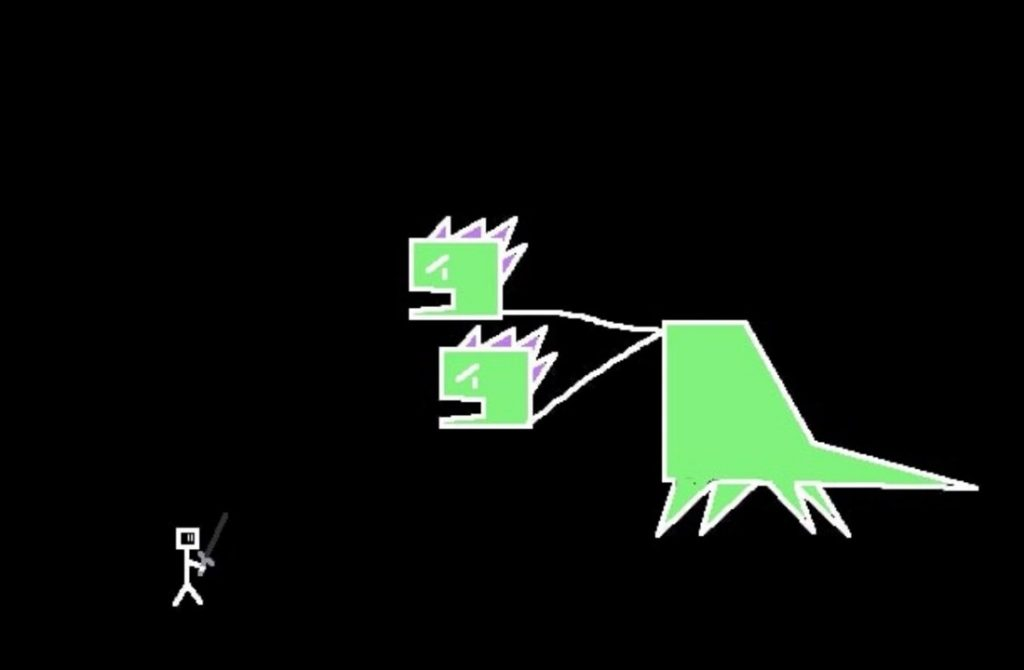Our blind hero stands before the two-headed Hydra. The chance of hitting the real head is 50% and the chance of hitting the false head is 50%.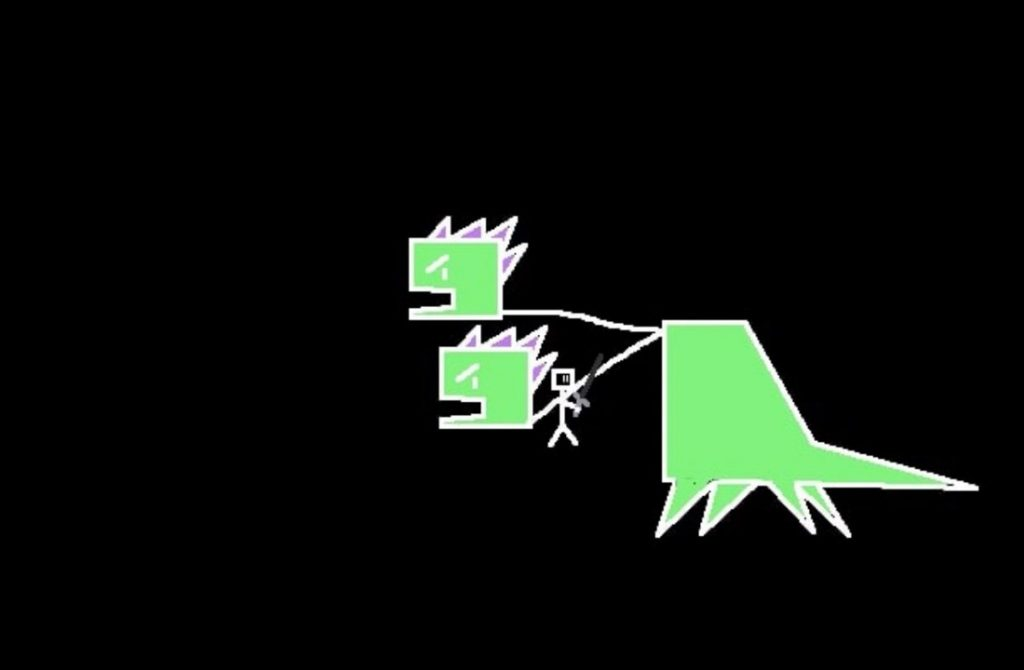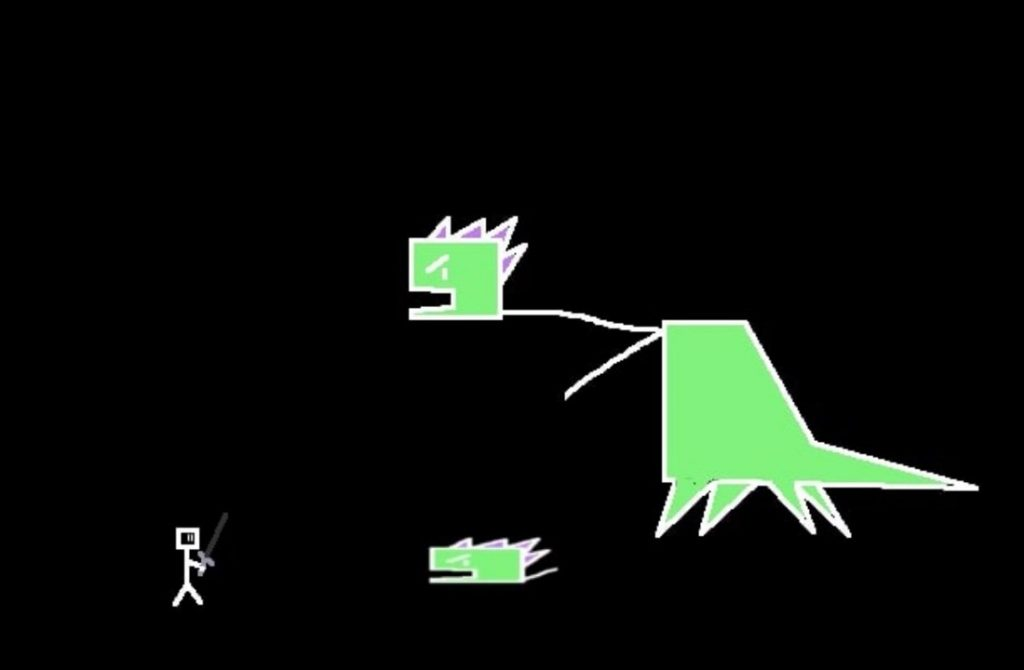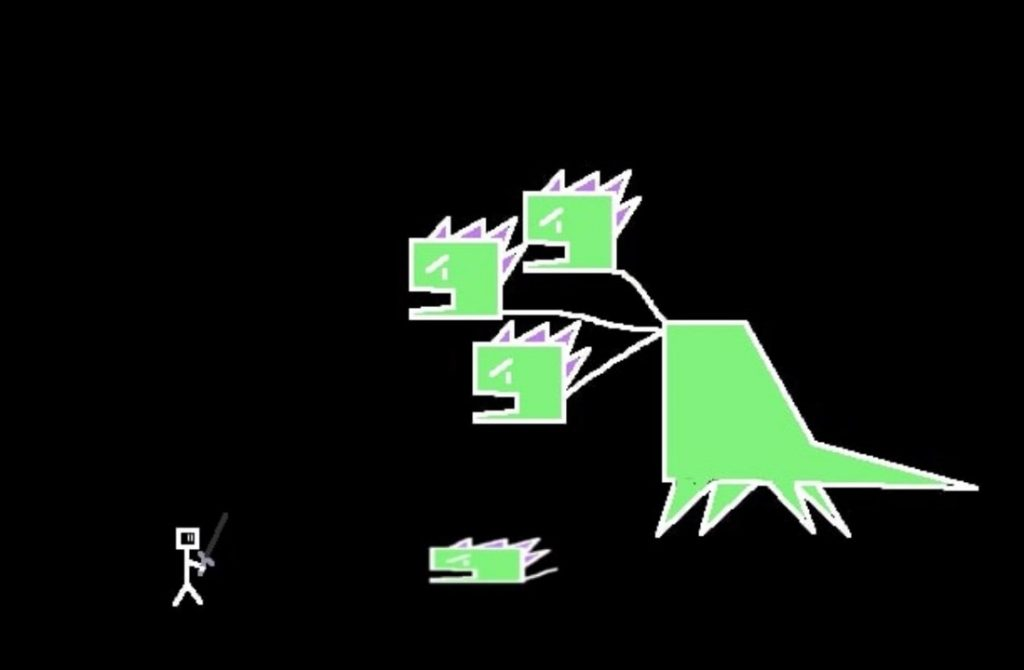But unfortunately, it was the false head. Then two new heads are born. Now your chance of hitting the real head is approximately 33% and the chance of hitting a false head is approximately 67%.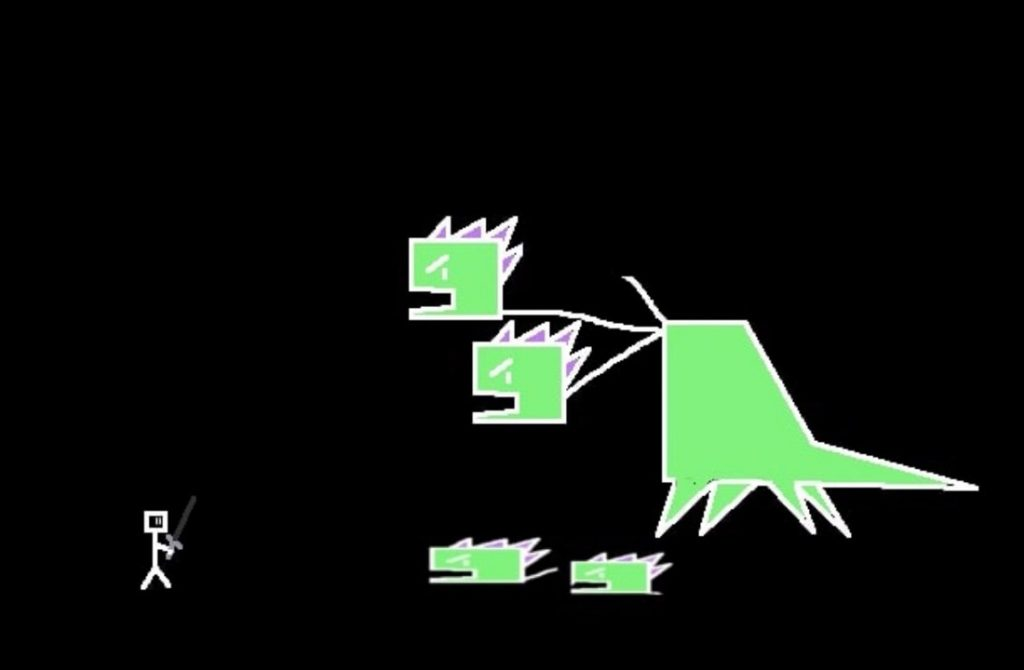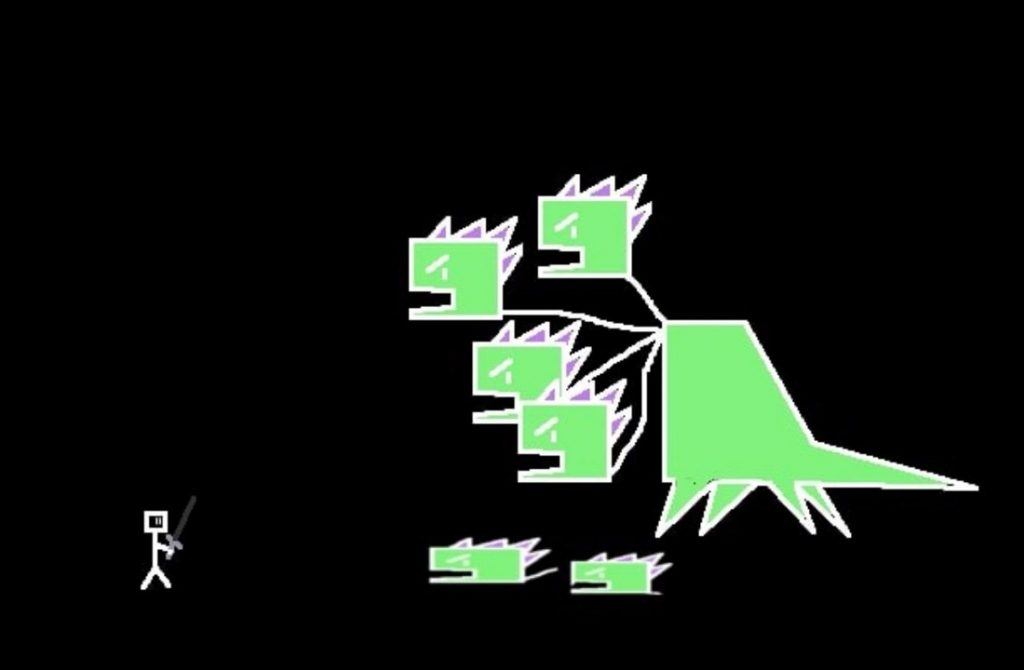But unfortunately, it was the false head. Then two new heads are born. Now your chance to hit the real head is 25% and the chance to hit a fake head is 75%.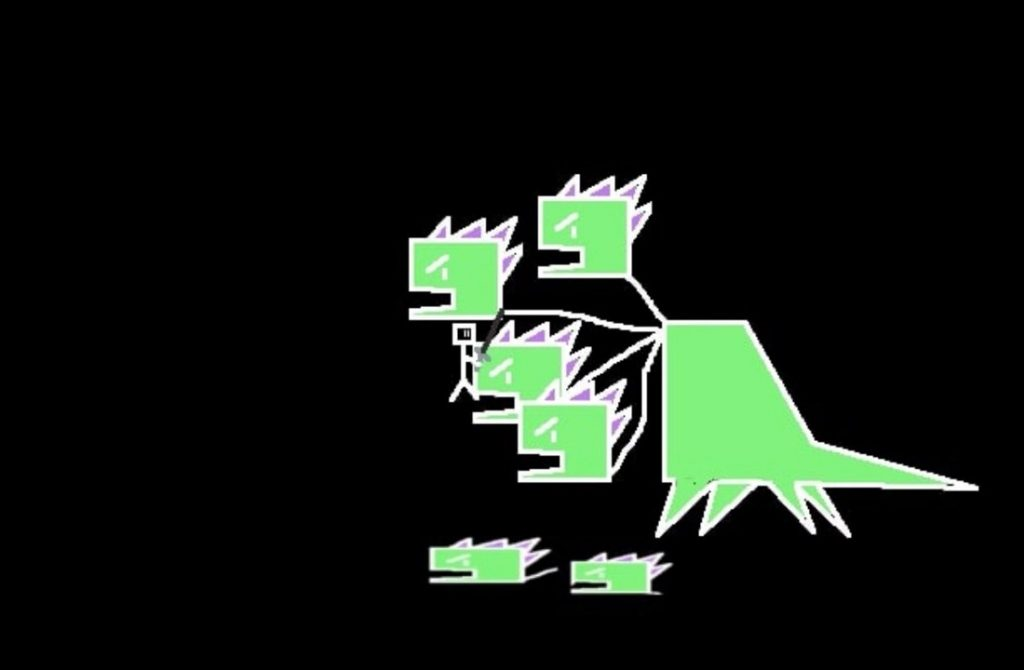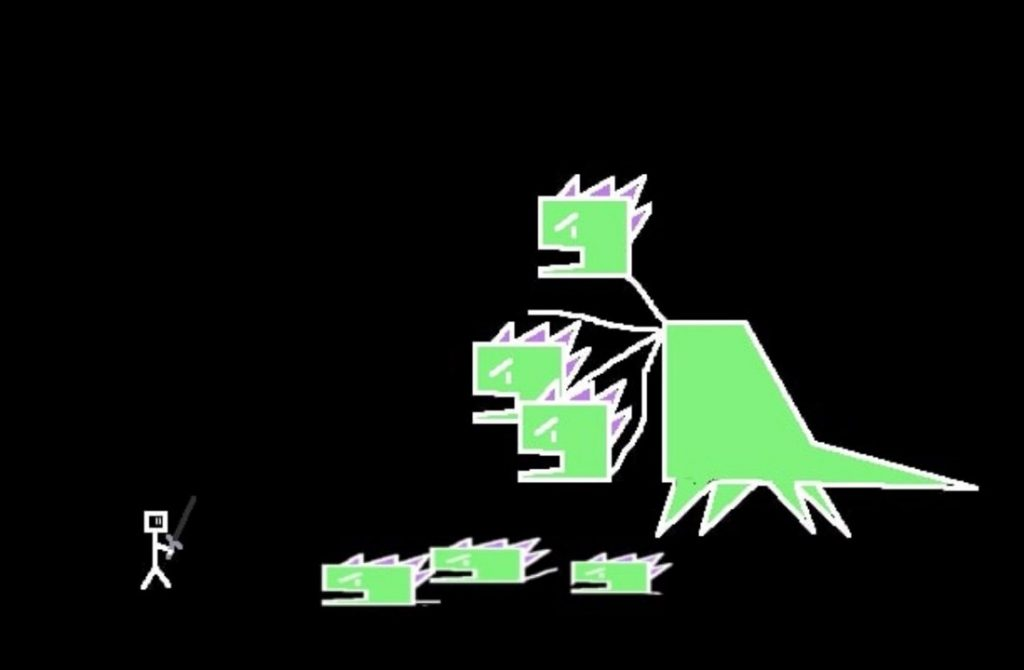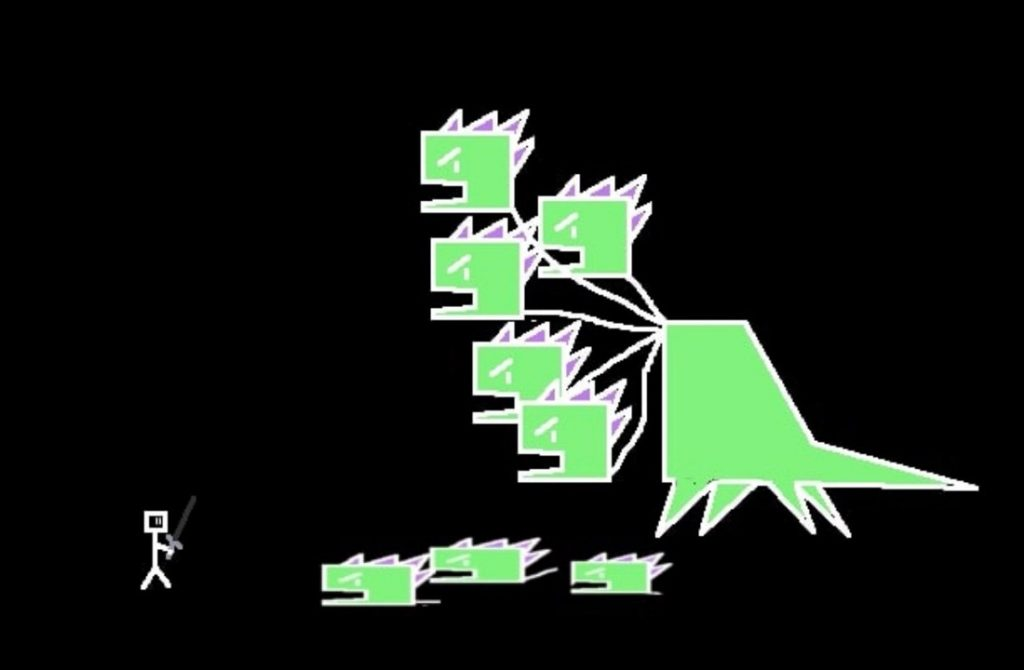But unfortunately, it was the false head. Then two new heads are born. Now your chance to hit the real head is 20% and the chance to hit a fake head is 80%. We will stop with just 5 heads, but in this problem, apparently the longer our hero takes to kill the Hydra, the more improbable the chance that he will hit the real head (given that the number of false heads increases). You may even think that killing it is a matter of luck, but unfortunately (for Hydra) the conditional probability tells us something else.

If we observe the chance of our hero defeating the Hydra with each new stroke, this probability will be 1/(number-total-heads), so for example, a Hydra with 100 heads, the chance of the hero hitting the real head will be 1%.

On the other hand (using conditional probability), let’s look at the Hydra’s chance of surviving each hit. For in the case of a Hydra that starts with two heads, it will only reach 100 heads, if the hero hits the wrong head 98 times.

For the Hydra to reach n+2 heads, the hero must have missed all the first n blows.

Analyzing then from the first of our hero’s mistakes, the chance of this Hydra surviving the first blow and reaching 3 heads is 50%.

However the chance of this Hydra surviving two strokes of the hero, will be the probability that it will survive the second stroke since it survived the first stroke. That is (50%).(67%), which gives 33%.

Similarly, the chance that this Hydra will survive three strokes of the hero, will be the probability that it will survive the third stroke since it survived the first and second strokes. That is (50%).(67%).(75%), which gives 25%.

Survive four strokes: probability of surviving the fourth stroke as it survived the first 3 strokes: (50%).(67%).(75%).(80%), which gives 20%.

We will only show the results for the following cases:

Survive five hits: 16%.

Survive six hits: 14%.

Survive seven hits: 12%.

Survive eight hits: 11%.

Survive nine hits: 10%.

Survive ten hits: 9%.

Survive 98 hits: 1%.

Survive 998 hits: 0.1%.

Thus, the chance of a Hydra in these conditions reaching 100 heads (surviving 98 hits) is approximately 1%. The chance of this same Hydra reaching 1000 heads is approximately 0.1%. In this way, we can see that as the number of attacks increases, the chance of the Hydra remaining alive decreases despite the number of heads increasing.

Although the chances of Hydra surviving will always increase (from the point of view of hero attacks), the probability of hitting a very large number of heads is getting closer and closer to 0%. That is, surely (sooner or later) the hero will land a blow to the real head and the fight will end.

You may be thinking that this only worked because our Hydra was very weak, if it were a “Super-Hydra” things could be different. Let’s suppose then that our hero is facing a “Super-Hydra” with N heads and with each false head destroyed, M heads appear. Any N and M natural numbers.

Likewise, your chance of surviving our hero’s first n attacks will be:

(N-1/N).(N-2+M/N-1+M).(N-3+2M/N-2+2M).(N-4+3M/N-3+3M) … (N-n+(n-1)M/N-(n-1)+(n-1).M).

Now it is difficult to perceive for any value of M and N, that as the number of attacks (n) increases, the chance of surviving the “Super-Hydra” will decrease. But note that the denominator is always greater than the quotient, so for each attack of the hero, the chance of the “Super-Hydra” to survive is less than 1. So when we multiply all these numbers less than 1, this product will always be in reduction, so the chance that the “Super-Hydra” will survive each new attack will always decrease, no matter the size of M and N.

For example, with M = 100 and N = 100.

After 10 attacks, the chance for the “Super-Hydra” to stay alive is 97%;

After 100 attacks, the chance of the “Super-Hydra” remaining alive is 95%.

This chance is slowly decreasing, but for a sufficiently large number, the chance of the “Super-Hydra” remaining alive will be very close to 0%.